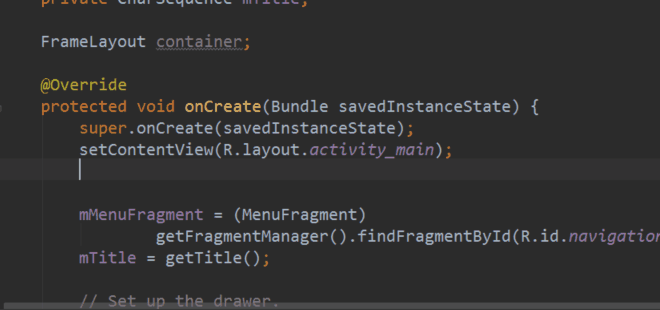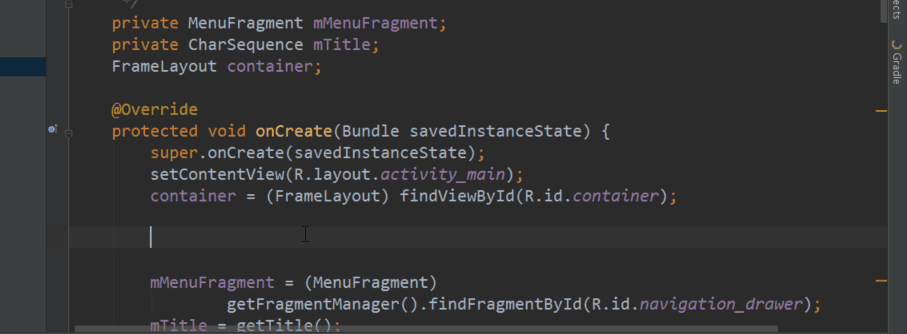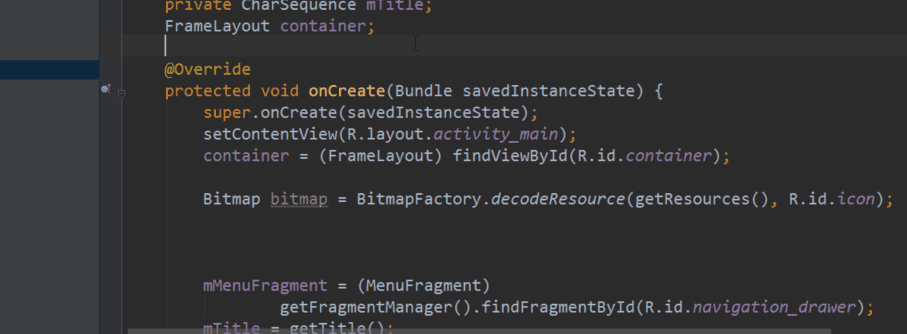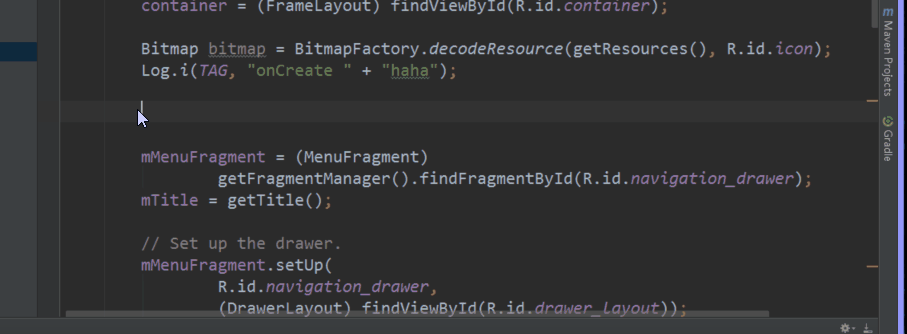Android Studio 之 Live Templates 高效利用

设置位置

• Settings - Editor - Live Templates

Live Template 语法

Name Expression Default value Skip if defined

Expression内有很多供使用的非常方便的函数，如className(), methodName()等等。设置Expression后别忘了勾选Skip if defined， 这样在使用的时候光标就不会再停留在这个变量处。

常用Android模板示例

findViewById 以下所有$cast$变量的expression值均为expectedType().

Name Template Text
fdv findViewById(R.id.$resId$);
fdvc ($cast$) findViewById(R.id.$resId$);
fdv_child $root$.findViewById(R.id.$resId$);
fdvc_child ($cast$) $root$.findViewById(R.id.$resId$);Bitmap初始化 $resource$ 设置defaultValue为"getResources()"

Name Template Text
bmp_res Bitmap $var$ = BitmapFactory.decodeResource($resource$, R.id.$resId$);Log

Name Template Text
tag private static final String TAG = “$class_name$”;
ld Log.d(TAG, “$method_name$” + $content$);
li Log.i(TAG, “$method_name$” + $content$);
le Log.e(TAG, “$method_name$” + $content$);
lv Log.v(TAG, “$method_name$” + $content$);
lw Log.w(TAG, “$method_name$” + $content$);For循环

Name Template Text
feach for ($i$ : $data$) {
$cursor$
}
fiter for ($i$=$start$; $i$<$end$; $i$++) {
$cursor$
}
fiter_with_init for (int $i$=$start$; $i$<$end$; $i$++) {
$cursor$
}Name Template Text
ci public static final int $VAR$ = $VALUE$;
cs public static final String $VAR$ = $VALUE$;